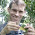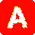## Thursday, January 31, 2013

### New version of pgls.Ives

I just posted a new version of the phytools function pgls.Ives, which is a function to fit the regression model of Ives et al. (2007) incorporating sampling error in the estimation of species means (e.g., here).

The main update in this version is that it can now accept individual data, rather than just species means, variances, and covariances. The form for the individual data is the same as fitBayes (e.g., here) - in other words, just long vectors with names equal to the species names. Obviously, some names will repeat - which is allowed in vectors.

There is nothing magic about the way this function works. It just takes the individual data, and then computes the within-species variances and covariances (which are themselves used to estimate within-species sampling variances and covariances). This is done using, for example, R base functions such as aggregate. For those in need of a quick primer, the following demo shows how aggregate can be used to compute within-species variances and covariances for a vector of values x containing individual values with names(x) being the species names, some of which repeat:
> # simulate tree & data
> tree<-pbtree(n=30)
> x<-fastBM(tree) # true means
> # individual values
> xe<-sampleFrom(xhat,randn=c(1,5))
> xe
t13         t13         t13         t13         t25
-1.83130612 -2.08011272 -1.99096486 -3.26950066 -1.17626558
t25         t26         t26         t20         t20
0.57125210 -1.90520230 -1.96856340         ...         ...

> # get species means
> xbar<-aggregate(xe,by=list(names(xe)),FUN=mean)
> xbar<-setNames(xbar[,2],xbar[,1])
> xbar
t1         t10         t11         t12         t13
-1.71666562 -3.92387486 -3.78403128 -1.82452852 -2.29297109
t14         t15         t16         t17         t18
-0.83735741 -0.66027704 -1.84528088        ...         ...

> # get species variances
> xvar<-aggregate(xe,by=list(names(xe)),FUN=var)
> xvar<-setNames(xvar[,2],xvar[,1])
> xvar
t1         t10         t11         t12         t13
0.319766112          NA 1.330431973 0.420867485 0.434420333
t14         t15         t16         t17         t18
0.910668871 0.985273462 0.560029491        ...         ...

Something that's apparent from the little simulation above, is that if there is not at least two samples for a particular species, then the species variance cannot be computed. In that case, the program merely assumes that those species have within-species variance equal to the mean within-species variance - but it will also spit a warning to that effect.

Computing the sampling covariance from individual data requires that the same individuals be sampled in both vectors. Thus, if the argument Cxy is not supplied, pgls.Ives will try to do it. If different individuals were used for x & y, then sampling covariance is probably negligible. In this case - if individual data is being supplied - then one should also give the function a named vector of zeroes for the argument Cxy to avoid any errors of spurious results.

Finally, here's a quick demo showing how to use individual data with pgls.Ives for both zero and non-zero error covariance:
> source("pgls.Ives.R")
>
> # simulate tree & data under the regression model
> tree<-pbtree(n=30)
> xhat<-fastBM(tree)
> yhat<-0.75*xhat+0.2*fastBM(tree)
>
> # simulate individual data for x & y
> # assuming Cxy!=0
> x<-sampleFrom(xhat,randn=c(1,10))
> n<-summary(as.factor(names(x)))
> y<-sampleFrom(yhat,n=n[names(yhat)])
>
> # fit model
> pgls.Ives(tree,x,y)
\$beta
 -1.193712  1.124268
\$sig2x
 0.5043893
\$sig2y
 0.219358
\$a
 3.111079 2.303972
\$logL
 -74.45021
\$convergence
 0
\$message
 "CONVERGENCE: REL_REDUCTION_OF_F <= FACTR*EPSMCH"

Warning messages:
1: Some species contain only one sample. Substituting mean variance.
2: Some species contain only one sample. Substituting mean variance.
3: Some species contain only one sample. Substituting mean variance.

Download the latest version of this function on the phytools page - or wait a bit and it will be in the next CRAN release.

1.Hi Liam,
I tried to run the script on real data. Usually, comparative data (both species-specific or individual-specific) are sorted in dataframes, in which species names are given in separate columns. With this structure the "aggregate" function does not work, returns with " Error in aggregate.data.frame(as.data.frame(x), ...) : arguments must have same length". Thus I have to calculate species-specific means and variances separately or restructure the data before running pgls.Ives. However, it would be much more convenient for the user, if you could change the command line for aggregate to take the species names from columns such as aggregate(my.data\$X, by=list(my.data\$species),FUN=mean).
Thanks.
All the best,
Laszlo Garamszegi

1.Hi Laszlo.

Thanks for the suggestion. Maybe I'll add that kind of input flexibility in the future at some point.

Here's a handy work-around that makes it very easy to run the function using the data structure you describe.

OK, let's say you have a data frame XX containing species names (with repeating species names for multiple samples per species), data vector x, and data vector y. Just run:

fit <- pgls.Ives(tree, x=setNames(XX\$x,XX\$species), y=setNames(XX\$y,XX\$species))

That's it!

All the best, Liam

2.Hi Liam,

This function allows incorporating sampling error in the estimation of the mean (i.e. standard error of the mean), but is it possible to incorporate the within species sample variance (i.e. the standard deviation)?

All the best,

Julien

3.Hi Liam,
I'm having trouble with the pgls.Ives function with my data. I have three samples for 7 species and one sample for 1 species for a total of 8 species. I've calculated the means and variances using aggregate so that I have a separate vector each for means and variances (and each entry is a sample, so there are up to three entries for the same species). The variance for one of the species is NA since there is only one sample.

Here is my command:
pgls.Ives(MetaboTree, Fl_PC1_bar, Lf_PC1_bar, Fl_PC1_var, Lf_PC1_var)
And here is the error:
Error in pd.solve(varcov, log.det = TRUE) : NA's in x

Any suggestions on where to look? When I remove the var variables and just execute:
pgls.Ives(IochromaMetaboTree, Fl_PC1_bar, Lf_PC1_bar)
Then it says:
Error in sig2x * C + diag(Mx) : non-conformable arrays

The length of the tree tips, means, and variances are all 8, and the is.na function doesn't reveal any apparent NAs.

Thanks!
Andrea

1.Well, it seems that I've fixed my own mistake. I was using the wrong vectors, working with species means and variances instead of letting each species entry exist individually in the vector. Cheers!

4.Hi Liam,
What is the best way to evaluate the significance of the regression fit in pgls.Ives? My output looks similar to your example above:
> (Kaemp_fit)
\$beta
 963.2129971 0.1879864

\$sig2x
 3560480732

\$sig2y
 426536077

\$a
 2326.028 1400.474

\$logL
 -161.5189

\$convergence
 0

\$message
 "CONVERGENCE: REL_REDUCTION_OF_F <= FACTR*EPSMCH"

But, when I use the summary(Kaemp_fit) command, it doesn't produce anything regarding the fit of the data:
Length Class Mode
beta 2 -none- numeric
sig2x 1 -none- numeric
sig2y 1 -none- numeric
a 2 -none- numeric
logL 1 -none- numeric
convergence 1 -none- numeric
message 1 -none- character

What would you recommend?
Thanks!
Andrea

5.Hi Liam,

Could you please clarify whether this function takes multiple dependent variables?

Thanks

Note: due to the very large amount of spam, all comments are now automatically submitted for moderation.